## General Chemistry

Learn the toughest concepts covered in Chemistry with step-by-step video tutorials and practice problems by world-class tutors

11. Bonding & Molecular Structure

# Formal Charge

Formal Charge is the charge given to an element within a molecule when assuming equal sharing of electrons between bonded elements.

Formal Charge
1
concept

## Formal Charge1m
Play a video:
now formal charge represents the charge given toe elements when assuming electrons are shared equally, regardless off electoral negativity. In reality, sometimes an element that's more electro negative will hold on to that electron within the electrons within the bond a little bit more tightly around themselves, which inflates or makes the electron clouds larger. But when it comes to formal charge, we don't take that into consideration. We assume that all atoms that are sharing electrons between each other they share them equally now with formal charge were introduced to terms such as bonding, electrons versus non bonding electrons. Bonding electrons are the electrons that do participate in bonding with other elements, and non bonding electrons are the electrons that don't participate and bonding with other elements with formal charge. We have our formal charge formula here. We'd say formal charge equals the valence electrons off that element that we're examining minus the bonds that it is making, plus the non bonding electrons that it possesses. We're going to say remember Valence electrons equals the group number off that element, and when we're talking about non bonding electrons, realized that we're gonna count them individually with formal charge when we find the formal charge of every element within a compound, we can calculate what's called the Net charge. The net charge is the overall charge. This is the sum off all formal charges within a compound. So now that we know the formal charge formula, click on to the next video on, Let's put it to practice with the given example.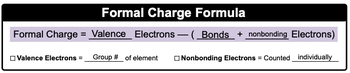Net Charge is the sum of individual formal charges within a compound.

2
example

## Formal Charge Example 156s
Play a video:
here we must determine the full charge of the nitrogen atom found within the ammonia molecule, which is NH three. All right, so we need formal charge, which is just going abbreviated. F C equals valence electrons, which is equal to the group number of the element. Nitrogen is a group five A. So it's five minus the bonds that it's making. It is making 123 bonds. Plus it's non bonding electrons, the electrons that are not participating in bonding, so they're counted individually. This would be one to non bonding electrons, so this would be five minus three plus two, so there'll be five minus five, so we'd have a formal charge of zero for the nitrogen atom within ammonia. This means that option C would be are correct answer.
3
Problem

Calculate the formal charges for each of the oxygen atoms within the nitrite ion, NO2.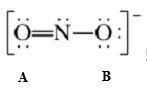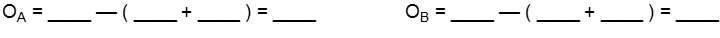4
Problem

Calculate the formal charge of the carbon atom within a carbon monoxide molecule.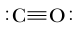a) –1

b) +2

c) 0

d) +

e) –2

5
Problem

Based on calculated formal charges, determine the overall charge (net charge) for the following compound.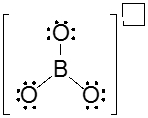a) –3

b) +4

c) +1

d) –1

e) +2

6
Problem

Which element within the thiocyanate ion possesses a negative charge?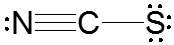a) C

b) S

c) N

d) All of them

e) None of them